# 基础知识

## 1. 参数法与非参数法

• 参数方法

根据先验知识假定模型服从某种分布，然后利用训练集估计出模型参数，也就弄清楚了整个模型。

假设了一个在整个输入空间上有效的模型，将问题归结为在样本上估计少量参数，(如：线性模型估计w，高斯分布估计mu和sigma)，参数学习方法假定了一个模型,当模型假定不成立或样本不是一个分组可能导致很大的误差。

例如感知器

• 非参数方法

不需要知道数据的概率分布，只需要假设：相似的输入具有相似的输出。基于记忆训练集，然后根据训练集预测。

非参数方法使用合适的距离度量相似性，对于输入样本，从训练集中找出它们的相似示例(输入样本的邻域)，并由相似的实例插值得到正确的输入。

例如kNN

## 2. 维度灾难

Curse of Dimensionality

## 3. 分类器性能指标

### 3.1 ROC 曲线

receiver operating characteristics 接收者操作特征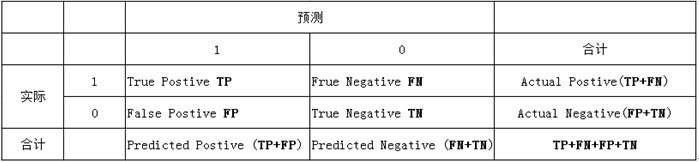1. 真正类率 (True Postive Rate)： $$TPR = \frac{TP}{TP+FN}$$， 代表分类器预测的正类中实际正实例占所有正实例的比例。Sensitivity

2. 负正类率 (False Postive Rate) ： $$FPR = \frac{FP}{FP+TN}$$，代表分类器预测的正类中实际负实例占所有负实例的比例。1-Specificity

3. 真负类率 (True Negative Rate) ： $$TNR = \frac{TN}{FP+TN}$$，代表分类器预测的负类中实际负实例占所有负实例的比例， $$TNR=1-FPR$$ 。Specificity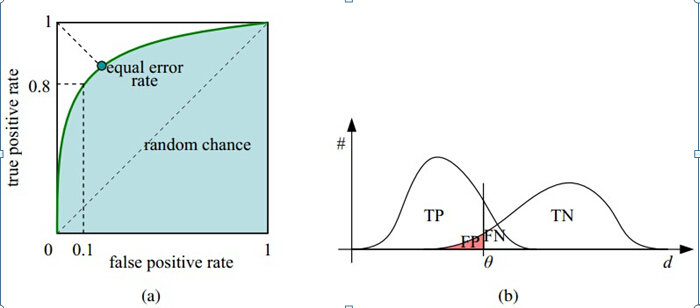$$TPR=1，FPR=0$$，即图中 $$(0,1)$$ 点，故ROC曲线越靠拢 $$(0,1)$$ 点，越偏离 45 度对角线越好，Sensitivity、Specificity 越大效果越好。

### 3.2 AUC

area under the curve

Roc 曲线下的面积，介于 0.1 和 1 之间。Auc 作为数值可以直观的评价分类器的好坏，值越大越好。

AUC 可以看做随机从正负样本中选取一对正负样本，其中正样本的得分大于负样本的概率！

AUC 对正负样本比例不敏感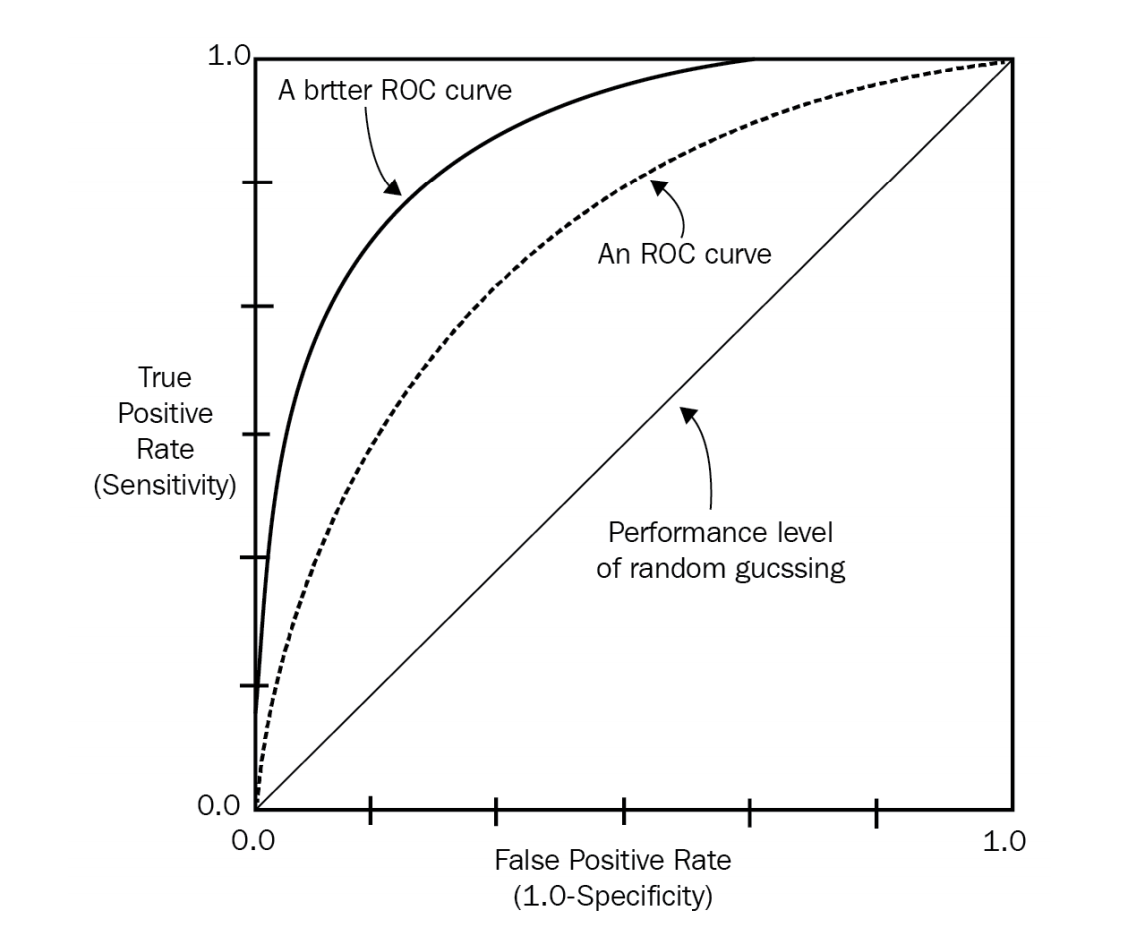# 方法

## 1. 梯度法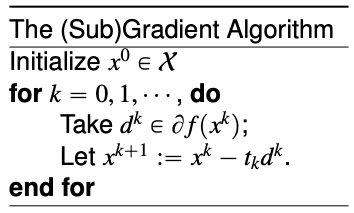## 1. 朴素贝叶斯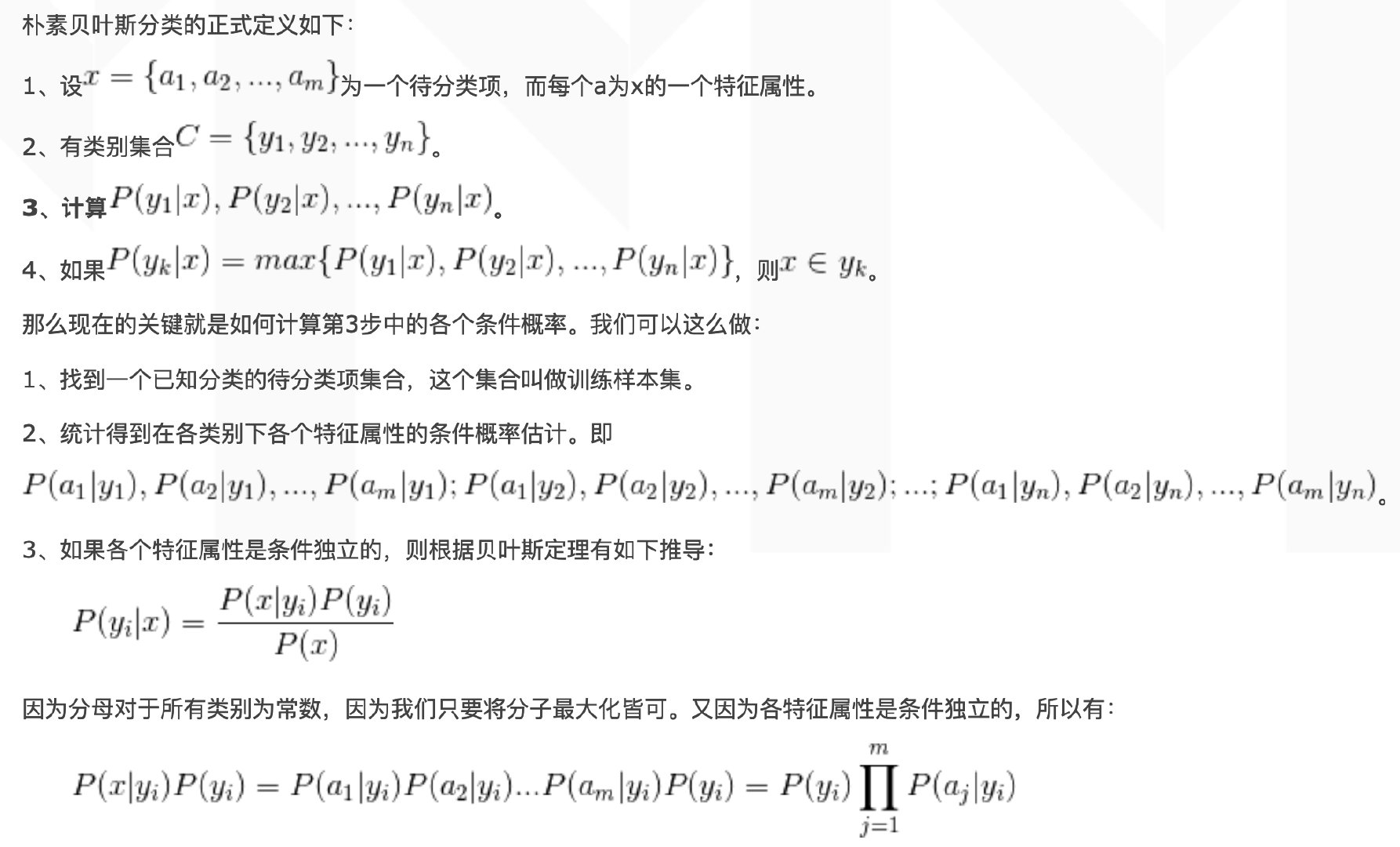![](/img/2019-03-25-ml-101.jpg)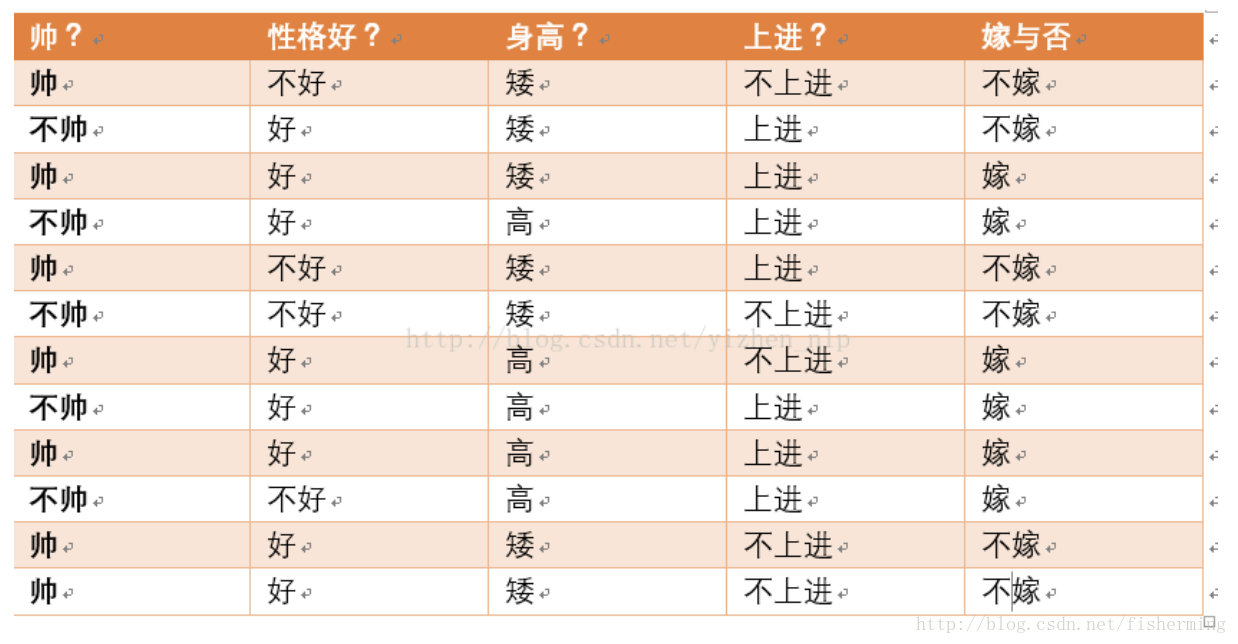• $$P(嫁 | 不帅、性格不好、矮、不上进)$$
• $$P(不嫁 | 不帅、性格不好、矮、不上进)$$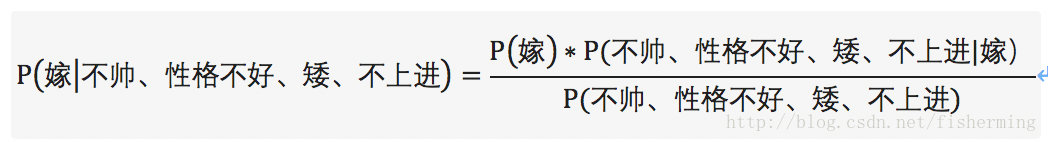$$P(不帅、性格不好、矮、不上进)=P(嫁)P(不帅|嫁)P(性格不好|嫁)P(矮|嫁)P(不上进|嫁)$$
$$+P(不嫁)P(不帅|不嫁)P(性格不好|不嫁)P(矮|不嫁)P(不上进|不嫁)$$

$$P(不帅、性格不好、矮、不上进|嫁)=P(不帅|嫁)P(性格不好|嫁)P(矮|嫁)P(不上进|嫁)$$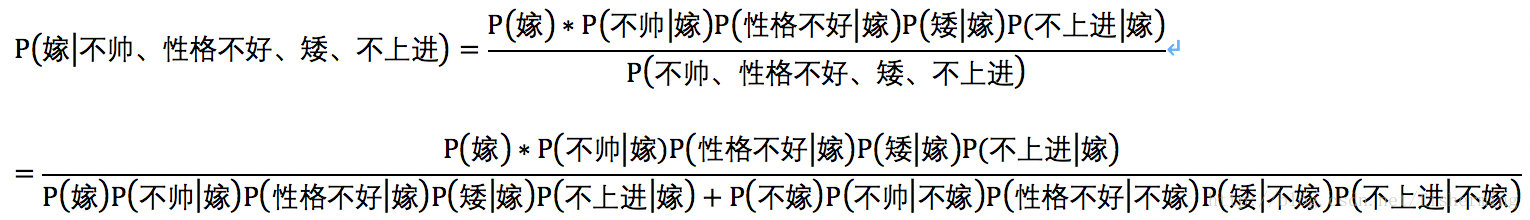$$P(嫁)P(不帅|嫁)P(性格不好|嫁)P(矮|嫁)P(不上进|嫁)=1/2 * 1/2 * 1/6 * 1/6 * 1/6=1/864$$

$$P(不嫁)P(不帅|不嫁)P(性格不好|不嫁)P(矮|不嫁)P(不上进|不嫁)=1/2 * 1/3 * 1/2 * 1* 2/3=1/18$$

$$P(嫁|不帅、性格不好、矮、不上进)=(1/864) / (1/864+1/18)=1/49=2.04%$$

$$P(不嫁|不帅、性格不好、矮、不上进)=(1/18) / (1/864+1/18)=48/49=97.96%$$

• 算法逻辑简单,易于实现（算法思路很简单，只要使用贝叶斯公式转化即可！）
• 分类过程中时空开销小（假设特征相互独立，只会涉及到二维存储）

• 朴素贝叶斯假设属性之间相互独立，这种假设在实际过程中往往是不成立的。在属性之间相关性越大，分类误差也就越大。